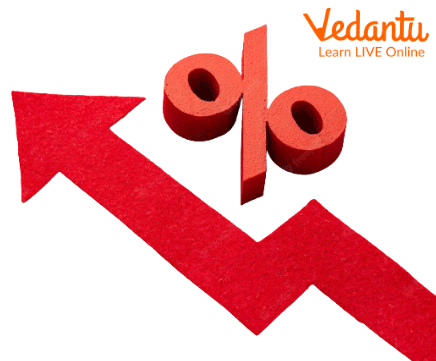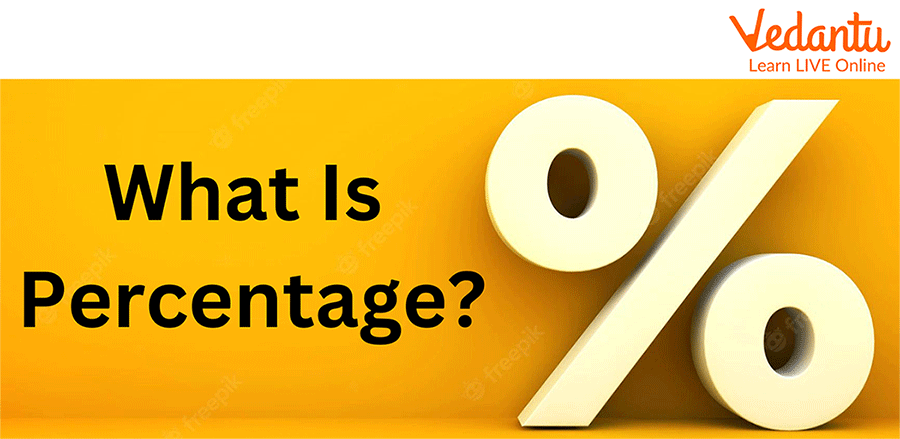Courses
Courses for Kids
Free study material
Free LIVE classes
More

# Percentage Word ProblemsLIVE
Join Vedantu’s FREE Mastercalss

## Introduction

Percentage is basically the ratio of the value of a particular quantity to its total value multiplied by 100. It is generally a way of expressing something as per 100. Further, in this article we will look at various scenarios for which we will find the percentage and learn the steps to solve word problems based on percentage.Percentage

### What is the Percentage?

A percentage is something expressed part per hundred. It is generally a ratio which is given in terms of a fraction of 100. The word percent is taken from the Latin term per centrum which means “by the hundred” . It is denoted by the symbol “$\%$”.What is Percentage

### How to Calculate the Percentage?

Percentage is generally calculated by expressing the value whose percentage is to be calculated and the total value as a ratio which is multiplied by 100. The formula for calculating percentage is as follows :

Percentage $= \frac{{value}}{{Total\,Value}} \times 100$

If in case, percentage of a number is to be calculated i.e. if % is given and the value of the quantity whose percentage is given with respect to the total is to be found we use -

For instance,${\rm{x\% }}$ of 450 $=$Y and we wish to calculate the value of Y, we can do so by

${\rm{Y}} = \frac{{\rm{X}}}{{100}} \times 450$

E.g. 20% of 800

So, $\frac{{20}}{{100}} \times 800 = 20 \times 8 = 160$

### Question and Answer based on Percentage

This section contains basic problems based on the concept of percentage which can be solved very easily.

1. Raju scored 81 out of 90 in mathematics. Convert his marks into percentages.

Ans. Percentage $=$ Value $/$Total Value $\times$100 $= \frac{{81}}{{90}} \times 100 = 9 \times 10 = 90\%$

1. In a class of 300 students, there are 75 girls. Calculate the percentage of boys in the class.

Ans. Number of boys $= 300 - 75 = 225$

Percentage of boys $= \frac{{225}}{{300}} \times 100 = 75\%$

1. Prince spent 75 $\%$ of the money he had to buy groceries. If he had 4000$with him initially, what amount did he spend ? Ans. Amount Spend $= \frac{{75}}{{100}} \times 4000 = 3000$$

1. Compute 65% of 680

Ans. 65% of 680 $= \frac{{65}}{{100}} \times 680 = 442$

### Word Problems based on Percentage

The word problem based on percentage will have some scenarios in which we have to understand the requirements in the given problems and accordingly apply the formula for percentage and find the value of the quantity asked to be found.

1.If a brass article contains 72% of copper. What amount of brass will be required to get 360g of copper?

Ans: Let the quantity of brass required be x g.

Therefore, 72% of x = 360g

$72\% \, \times \,x = 360g$

$0.72 \times x = 360g$

Therefore, $x = \frac{{360}}{{0.72}} = 500g$

So, 500 g of brass is required to get 360g of copper.

2.Karishma appeared for a quiz in which she got 25 answers correct and 15 answers incorrect. What is the percentage of questions that she appeared correctly ?

Ans : Here, in this case 25 answers were correct and 15 were incorrect.

Therefore, total questions $= 25 + 15 = 40$

So, Percentage of correct questions $= \frac{{25}}{{40}} \times 100 = 62.5\%$

Therefore, 62.5$\%$ of the questions were answered correctly by Karishma.

### Solved Examples :

1. What is 30$\%$ of 450?

Solution : 30 % of 450 $= \frac{{30}}{{100}} \times 450 = 0.3 \times 450 = 135$

Therefore, 30% of 450 is 135.

2. The cost price of a bag is 1500 and the selling price of the same bag is 2100. At what profit is the article sold? What is the profit percentage?

Solution : As , Profit = Selling price - Cost price

Therefore, Profit earned on selling the bag $= 2100 - 1500 = 600$

So, Profit Percentage $= \frac{{600}}{{1500}} \times 100 = 40\%$

The seller earned 40$\%$ profit on selling the bag.

3. Arun sells an object to Benny at a profit of 15%, Benny sells that object to Chandan for ₹1012 and makes a profit of 10%. At what cost did Arun purchase the object?

Solution: Let the actual cost price at which Arun bought the object be x

When Arun sells the object to Benny

Profit % = 15%

∴ selling price of object $= \frac{{100 + 15}}{{100}} \times x = 1.15x$

Now, this cost price of the object for Benny

When Benny sells the object to Chandan

Selling Price = ₹1012

Profit % = 10%

∴ Selling price = $= \frac{{100 + 10}}{{100}} \times 1.15x$

$\Rightarrow 1012 = \frac{{100 + 10}}{{100}} \times 1.15x$

$\Rightarrow x = \frac{{1012 \times 1000}}{{11 \times 115}}$

= ₹800

Therefore, the price at which Arun bought the object is ₹800.

4. In an inter school aptitude test, 200 students appeared. Out of these students; 20 % got A grade, 50 % got B grade and the remaining got C grade. Assuming that no student got a D grade; find the number of students who got a C grade .

Solution : The number of students with A grade $=$ 20$\%$ of 200

$= \frac{{20}}{{100}} \times 200 = \frac{{4000}}{{100}}$

$= 40$

And, the number of students with B grade $=$ 50 $\%$ of 200

$= \frac{{50}}{{100}} \times 200$

$= \frac{{10000}}{{100}} = 100$

Therefore, the number of students who got C grade $= 200 - [40 + 100]$

$= 200 - 140 = 60$

So, 60 students scored C grade.

### Conclusion:

Thus, we can say that percentage is a fraction of something expressed as part of 100. So, after understanding the method of calculating percentage and applying it according to the scenario we can easily solve the word problems based on percentage.

Last updated date: 30th Sep 2023
Total views: 81.9k
Views today: 1.81k

## FAQs on Percentage Word Problems

1. Can the percentage be negative?

No, percentage can never be negative.

2. Is 0% of something valid ?

Yes, it is valid. For instance, if an alloy contains 0% of a certain element it simply means that the element is not present in that alloy.

Example: $1250 \times 0\% = 0$

3. Can percentage be represented in decimals?

Yes, percentage can be represented in decimals. For example, 25% of something means $\frac{{25}}{{100}} = 0.25$$\frac{{25}}{{100}} = 0.25$